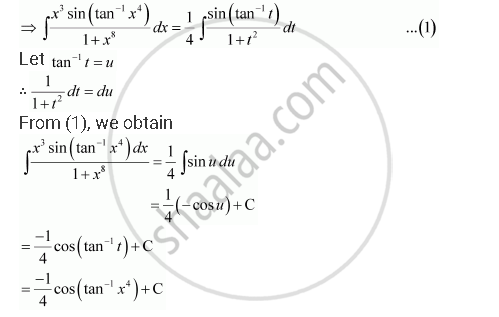Share
Notifications

View all notifications
Books Shortlist
Your shortlist is empty

# Solution for Integrate the Functions in (X^3 Sin(Tan^(-1) X^4))/(1 + X^8) - CBSE (Science) Class 12 - Mathematics

Login
Create free account

Forgot password?
ConceptMethods of Integration - Integration by Substitution

#### Question

Integrate the functions in (x^3 sin(tan^(-1) x^4))/(1 + x^8)

#### Solution

Let x4 = t

∴ 4x3 dx = dtIs there an error in this question or solution?

#### Video TutorialsVIEW ALL 

Solution Integrate the Functions in (X^3 Sin(Tan^(-1) X^4))/(1 + X^8) Concept: Methods of Integration - Integration by Substitution.
S